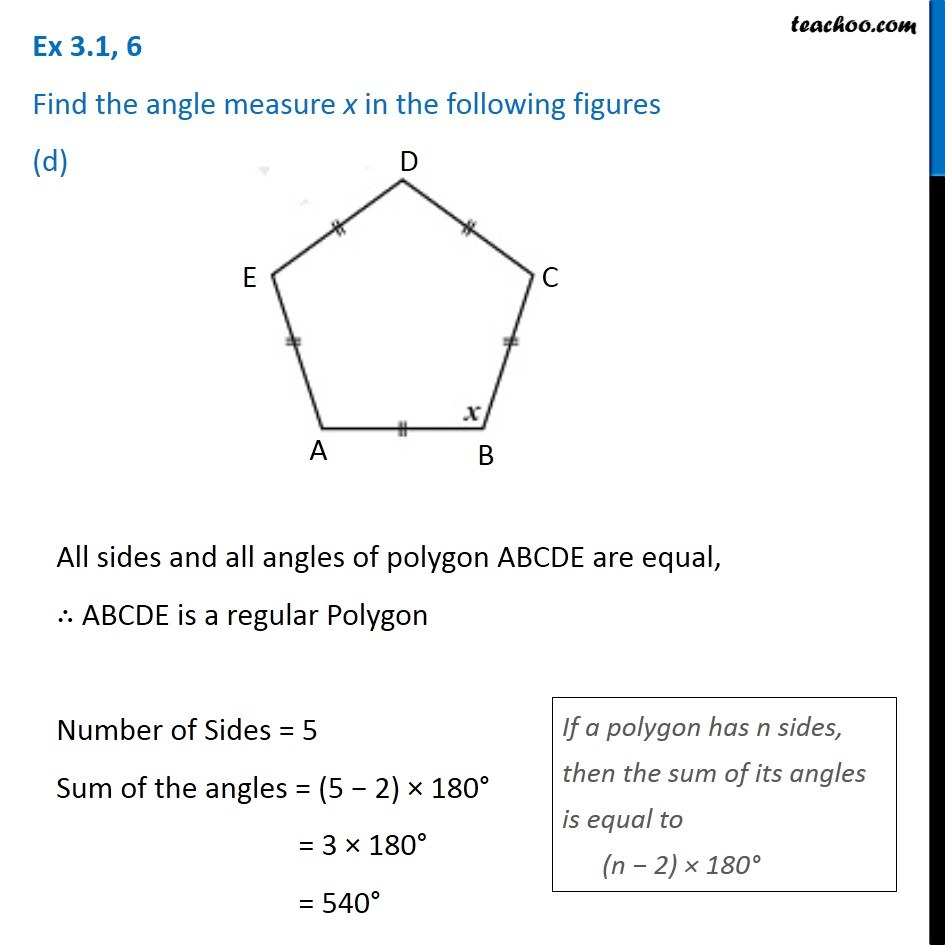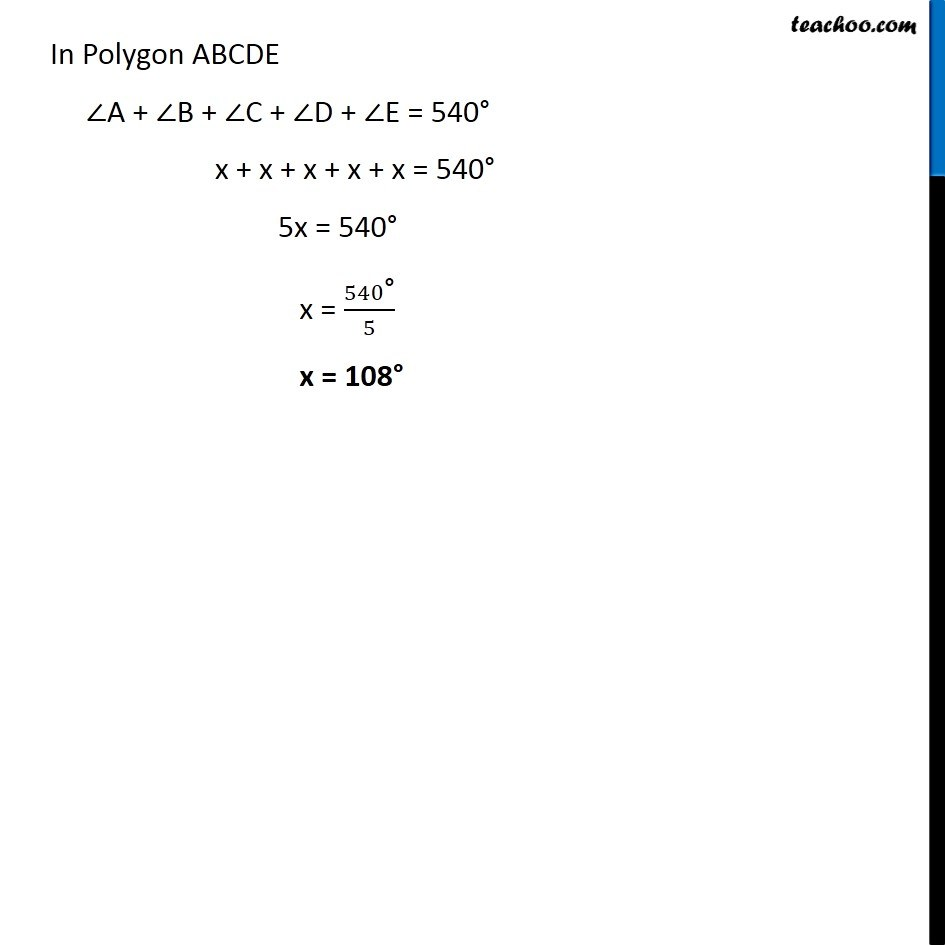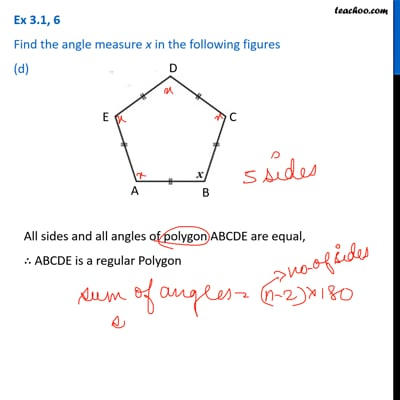Ex 3.1

Chapter 3 Class 8 Understanding Quadrilaterals
Serial order wiseThis video is only available for Teachoo black users

Learn in your speed, with individual attention - Teachoo Maths 1-on-1 Class

### Transcript

Ex 3.1, 6 Find the angle measure x in the following figures (d) All sides and all angles of polygon ABCDE are equal, ∴ ABCDE is a regular Polygon Number of Sides = 5 Sum of the angles = (5 − 2) × 180° = 3 × 180° = 540° If a polygon has n sides, then the sum of its angles is equal to (n − 2) × 180° In Polygon ABCDE ∠A + ∠B + ∠C + ∠D + ∠E = 540° x + x + x + x + x = 540° 5x = 540° x = 540"°" /5 x = 108°